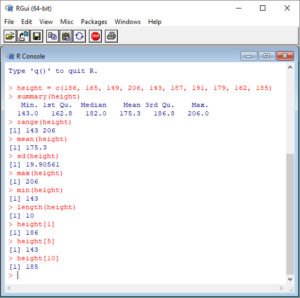Many of you have heard of R (the R statistics language and environment for scientific and statistical computing and graphics). Perhaps you know that it uses command line input rather than pull-down menus. Perhaps you feel that this makes R hard to use and somewhat intimidating!

Indeed, R has a longer learning curve than other systems, but don’t let that put you off! Once you master the syntax, you have control of an immensely powerful analytic tool. Actually, much of the syntax is not all that difficult. Don’t believe me? To prove it, let’s look at some syntax for providing summary statistics on a continuous variable. First install R by going to the following website:

http://cran.r-project.org/bin/windows/base/

Download R 3.5.0 for Windows and then install R as instructed in this blog post.

OK, so you have successfully downloaded and installed R. Now you should have the R icon on your desktop.Click this icon to open R.Now for a simple exercise. Let’s take height to be a variable that describes the heights (in cm) of ten people. Copy and paste the following code to the R command line to create this variable.

`height = c(186, 165, 149, 206, 143, 187, 191, 179, 162, 185)`

The variable is now stored in the R workspace. To view this variable, enter:

`height`

Now, enter the following code at the command line and hit return after each piece of code. You will be surprised how easy this really is!

```summary(height) range(height) mean(height) sd(height) max(height) min(height) length(height) height `````` height```
`height`
So – it really wasn’t that difficult after all. More about R later.
David

#### Annex: R codes used

```# Creating the height variable
height = c(186, 165, 149, 206, 143, 187, 191, 179, 162, 185)

# Descriptive statistics of the height variable
summary(height)

# Range of values (minimum, maximum) of the height variable
range(height)

# Mean value of the height variable
mean(height)

# Standard deviation of the height variable
sd(height)

# Maximum value of the height variable
max(height)

# Minimal value of the height variable
min(height)

# Length, i.e. number of observations of the height variable
length(height)

# Value of the first observation of the height variable
height

# Value of the fifth observation of the height variable
height

# Value of the tenths, i.e. last observation of the height variable
height
```

Screenshot of the R console with all results: